# Air thermal

Imagine that a unit of air rises at 3000 meters high, if temperature decreases 6 degrees celcius for every 1000 meter, what will be its temperature at 1400 meters, 2000 meters, 2500 meters and when it reaches the 3000 meter elevation. Starting temperature of air is 35°C.

t1 =  26.6 °C
t2 =  23 °C
t3 =  20 °C
t4 =  17 °C

### Step-by-step explanation: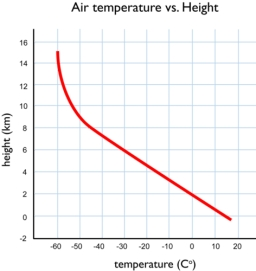Did you find an error or inaccuracy? Feel free to write us. Thank you!## Related math problems and questions:

• Imagine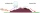Imagine that a unit of air at a temperature of 25°C rises up a mountain range that is 3,000 meters high on the windward side and which descends to 1,200 meters on the leeward side, assuming that the air will remain dry what will its temperature when it cr
• Electrics - conductor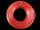The wire is 106 meters long at 0 °C and at every temperature increase of 1 °C the length increases by 0.15 mm per 1 m length of wire. Determine a function which represents the overall length of the wire as a function of temperature. What is the length of
• Now isNow is 5°C morning. What will the temperature be if i. It rises by 12°C ii. It falls by 15°C
• Bath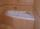In the bath is 30 liters of hot water. Then added 36 liters of cold water at temperature of 19 °C decreased temperature of water to 41 °C. What was the initial temperature of the hot water?
• Latent heat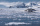How much heat is needed to take from 100 g of water at 20°C to cool to ice at -18°C? Mass heat capacity c (ice) = 21kJ/kg / °C; c (water) = 4.19 KJ/kg / °C and the mass group heat of solidification of water is l = 334kJ/kg
• ThereThere is 5 l of water with a temperature of 20°C in the bucket. We add 3 liters of hot water. The resulting temperature will be 30°C. What was the temperature of the hot water?
• Temperature calculus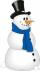Calculate the percentage of temperature increase if changed from 18°C to 33°C?
• Heat transferWe placed a lead object weighing 0.4 kg and 250°C in 0.4 L water. What was the initial temperature of water t2 if the object's temperature and the water after reaching equilibrium was 35°C? We assume that the heat exchange occurred only between the lead o
• WeatherWeather. At 5:00 am, the temperature was 28°C, and at noontime, it rose by 9°C. By 4:00 pm, it dropped 3°C, and by nighttime, it was 4°C less. What is the room temperature this time?
• The temperature 13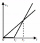The temperature in Toronto at noon during a winter day measured 4°C. The temperature started dropping 2° every hour. Which inequality can be used to find the number of hours, x, after which the temperature will measure below -3°C?
• Mine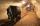What is temperature in the mine at a depth of 1160 m, where at depth 9 m is 11°C and every 100 m, the temperature increases by 0.7°C?
• Water temperaturesTo 25 liters of water at 50 °C we add 15 liters of water at a different temperature. How many °C should the water be colder than 50 °C so that 40 liters of the water obtained has a temperature 42.5 °C?
• Mixing waterWe should fill the 30-liter container with water at 60 degrees Celsius. How many liters of water 80 degrees C hot, and how many liters of water 20 degrees Celsius warm we have to mix?
• Milk mixing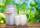You have 12 liters of milk with a temperature of 20°C. How much milk at 80°C do you have to add to have milk at 30°C?
• The bathThe bath has water with a volume of 220 l and a temperature of 65°C. Determine the mass m2 of water at 15°C to be poured so that the resulting temperature of the water in the bath is 40°C. We neglect the heat exchange between water and the environment. T
• What power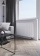What power should the radiator have in a room 5x4 meters with a height of 3 meters? Hint: Calculate 35 watts per meter ^ 3 in case you need 22 degrees in the room but you need about 24 degrees in the bathroom. It's an old heating cheat, but it will defini
• Thermometer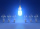Immerse a thermometer with a heat capacity of 2.0 J. K-1 in water weighing 67.0 grams. Before immersion in water, the thermometer showed a temperature of 17.8 degrees Celsius. After reaching equilibrium, the temperature is 32.4 degrees. What was the water